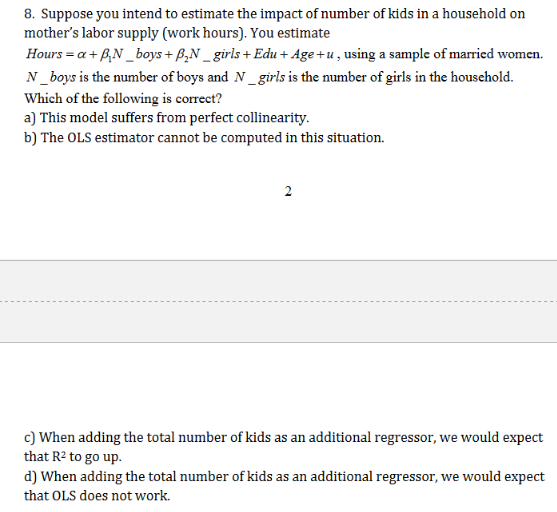# 8. Suppose you intend to estimate the impact of number of kids in a household onmother's labor supply (work hours). You estimateHours a+ pN_boys+ P,N_girls+ Edu+ Age+u,using a sample of married womenN_boys is the number of boys and N_girls is the number of girls in the household.Which of the following is correct?a) This model suffers from perfect collinearityb) The OLS estimator cannot be computed in this situation2c) When adding the total number of kids as an additional regressor, we would expectthat R2 to go upd) When adding the total number of kids as an additional regressor, we would expectthat OLS does not work.

Question
45 viewshelp_outlineImage Transcriptionclose8. Suppose you intend to estimate the impact of number of kids in a household on mother's labor supply (work hours). You estimate Hours a+ pN_boys+ P,N_girls+ Edu+ Age+u,using a sample of married women N_boys is the number of boys and N_girls is the number of girls in the household. Which of the following is correct? a) This model suffers from perfect collinearity b) The OLS estimator cannot be computed in this situation 2 c) When adding the total number of kids as an additional regressor, we would expect that R2 to go up d) When adding the total number of kids as an additional regressor, we would expect that OLS does not work. fullscreen
check_circle

Step 1

When you add another independent variable in the equation R2 will always increase. So here we are adding another variable i.e. No of kids in the...

### Want to see the full answer?

See Solution

#### Want to see this answer and more?

Solutions are written by subject experts who are available 24/7. Questions are typically answered within 1 hour.*

See Solution
*Response times may vary by subject and question.
Tagged in

### Other